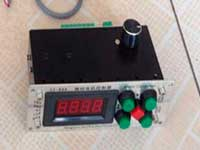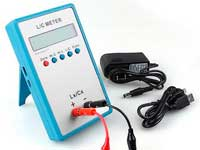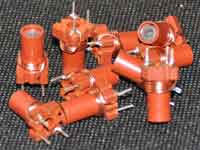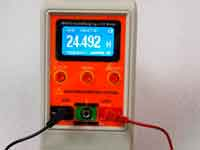RF coil with an arbitrary winding pitch - 4.0 out of 5 based on 3 votes
Rating 4.00 (3 Votes)

Calculation of the single layer RF coil with an arbitrary winding pitch.For the calculation of the single-layer inductors can be used Maxwell's model, then each turn represents as a circular ring. The Lorentz model is an attempt to avoid this simplification, however, and it works with good accuracy only if the turns are close to each other and in the end, both models are not suitable for the calculation of the helical coils with a large winding pitch.

How to solve the problem of calculation of such coils? First, using a semiempirical correction formula. Is the easy path.

Single-layer coil with winding pitch can be calculated as a singlelayer coil with close-winding. Only the amendment to the resulting inductance is added:where:

• ΔL is the amendment to the inductance of the close-winding coil (always negative) (µH)
• N - number of turns
• D - diameter of winding (mm)
• d - diameter of wire (mm)
• p - winding pitch (distance between centers of neighboring turns = the gap between the turns + wire diameter) (mm)

The formula gives an error of about ±5-10% when the number of turns of at least 5 and the winding pitch of not more than two diameters of the wire. The formula used in previous versions of the program (prior to v9.0)Now Coil32 uses a more advanced method of calculation of the single layer coil with an arbitrary pitch of winding with any number of turns.

In 1926 Chester Snow successfully tried to create a realistic model of the coil with a pitch of winding. He developed a mathematics method of calculating the mutual inductance between two infinitely thin helical conductors. Using the concept of "geometric mean distance", which introduced J.C. Maxwell, it is possible to calculate the self-inductance of the helical coil. However, the formula of C. Snow is inaccurate, particularly in the limiting cases of a single turn of the helix, and when the helix with a endless pitch of winding degenerates into a straight wire - formula gives the wrong result. In 2012, Robert Weaver, based on a Snow's method and based on the integral of F. Neumann led his formula for calculating coil with an arbitrary pitch:All designations and dimensions are given in cylindrical coordinates, the details in the original work.

In his work, Robert Weaver leads a method for solving this integral using numerical algorithm, based on Simpson's rule and on Newton - Raphson method and provides the programming algorithm, which is used in Coil32 v11.7.0.900 for Windows, in cross-platform app Coil64 and in Android version with slight modifications, allowing to accelerate the iteration. Earlier this calculation method was used in the plugin Precise Helix, now it's in the main code of the program.

Сomments from anonymous guests are enabled with moderation.

Coil Winding MachineUS \$187.53
The system consists of individual components including a controller, a stepping motor, a foot switch, power adapter and output shaft. This design makes it very easy for you to integrate the system into your own project.

LC Meter LC200AUS \$37.99
Measuring Capacitance Range:
0.01 pF - 100mF
Measuring Inductance Range:
0.001 uH - 100H
Measurement accuracy:1%.

RF InductorsUS \$6.43
Variable Ferrite Core Inductor coil set 3.5Turns 25-100MHZ

Auto Ranging LCR MeterUS \$63.07
Meter can measure 0.00pF to 100.00mF capacitance, 0.000uH to 100.00H inductance and 0.00? to 10.000M? resistance. Very suitable for small value capacitor and inductor measurement.